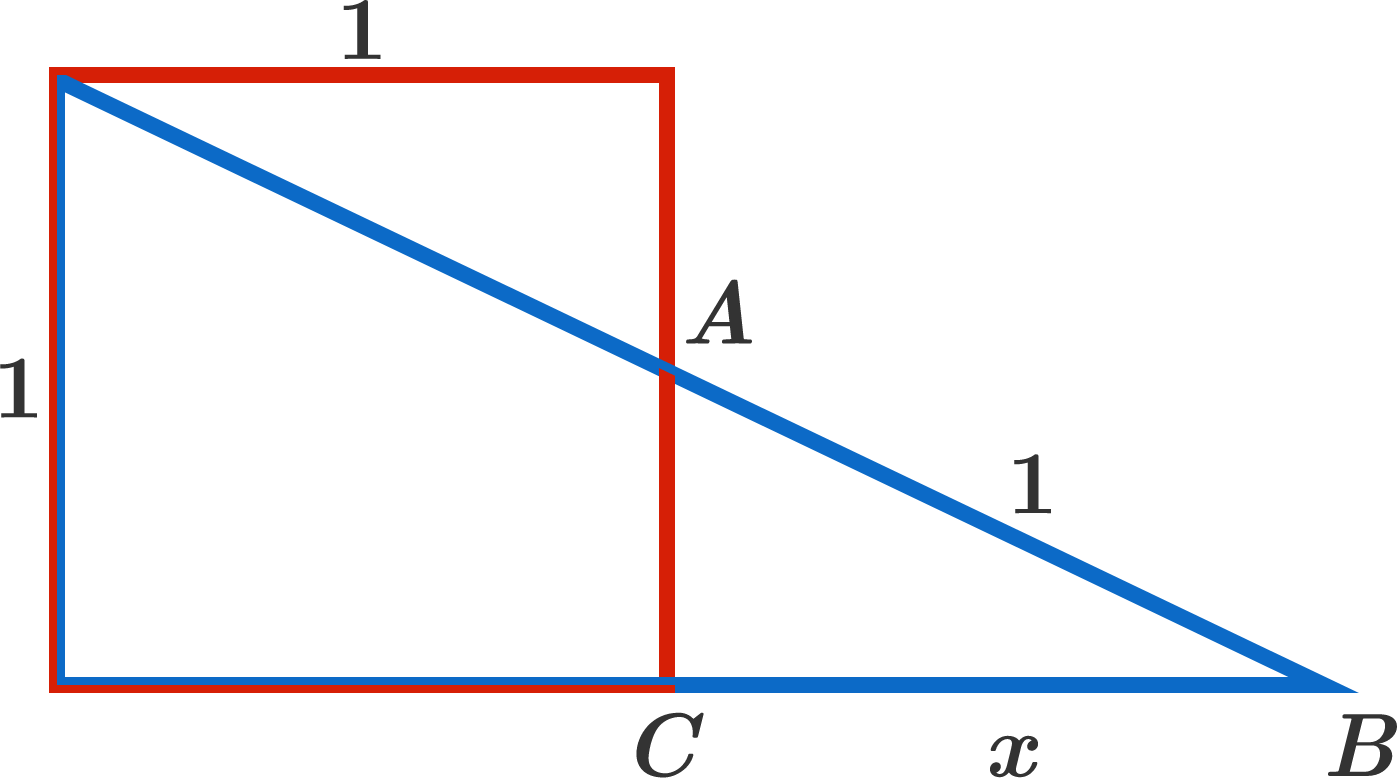# Trigonometry or Square completing?

Geometry Level 3In the diagram above, the blue right triangle is partially overlapping the $1\times1$ red square, sharing a corner. If $\overline{AB}=1$ and $\overline{BC}=x,$ find $x$ to 3 decimal places.

×

Problem Loading...

Note Loading...

Set Loading...